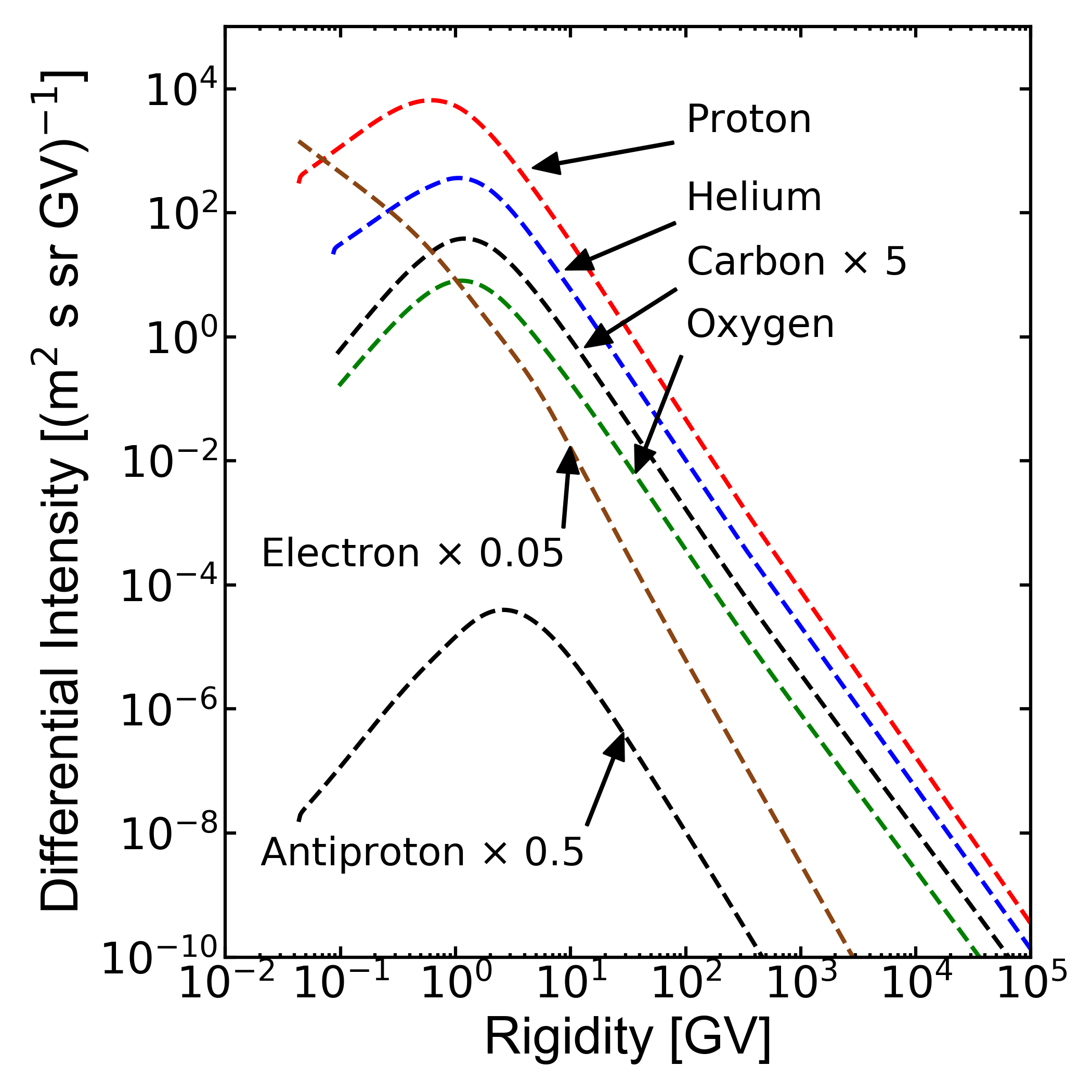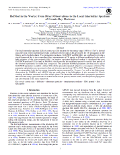## GALPROP obtained LIS using modulated spectra from HelModThe local interstellar spectra (LIS) are input cosmic ray intensities for any modulation models. Fluxes are assumed isotropically distributed at heliosphere boundary, in a steady-state configuration. The LIS is usually computed using numerical model accounting for the propagation in galaxy. The heliosphere modulation is the added using, e.g.,the modulation module available here for on line use or download.

Recently, Della Torre et al. 2017, Boschini et al 2017 deduced LIS's for protons, helium nuclei and antiprotons using the most recent experimental results combined with GALPROP model for propagation in galaxy and HelMod for the propagation in heliosphere.

Successive work extend the available sets of LIS's. Boschini et al 2018b, using the same procedure, deduced LIS's  for Carbon- and Oxygen-nuclei, while Boschini et al 2018c deduced LIS for galactic electrons.

HelMod and GALPROP were combined to provide a single framework and run to reproduce a comprehensive set of observations of CR species collected in different time periods, from 1997 up to 2015.

The numerical table of computed LIS is available as supplementary material of Boschini et al 2017Boschini et al 2018b, and Boschini et al 2018c.

### Antiproton, Proton and helium nuclei LIS

In Della Torre et al. 2017, Boschini et al 2018,2017 authors proposed an analytical expression for proton and helium nuclei LIS's as a function of the rigidity:
\begin{equation}
J_{LIS}(P)\times R^{2.7} =\left\{
\begin{array}{ll}
\sum_{i=0}^5 a_i P^{i}, &R\le1\ {\rm GV},\\
b + \frac{c}{R} + \frac{d_1}{d_2+R} + \frac{e_1}{e_2+R} + \frac{f_1}{f_2+R} + g R, & R>1 \ {\rm GV},
\end{array}
\right.
\end{equation}
where $$a_i, b, c, d_i, e_i, f_i, g$$ are the numerical coefficients summarized as follow:

The accuracy of the low-energy expression is 2% in the range $$0.2$$ GV$$< R < 1$$ GV for the proton LIS. The high-energy part reproduces the numerical proton LIS calculated with GALPROP with an accuracy of $$\sim$$9\% for $$0.45$$ GV $$< R < 1$$ GV (i.e., $$E_{\rm kin}>0.11$$ GeV), where the constraints from Voyager 1 are wider than 10%, and of $$2$$% for $$R>1$$ GV. The discrepancies with respect to AMS-02 data at higher energies expressed in standard deviations are virtually zero, with $$0.5\sigma$$ around $$1-2$$ GV at most. In the case of Helium, the low-energy expression is valid in the range of $$0.15\ {\rm GV} < R < 2$$ GV, i.e., approximately between 3 MeV/nucleon and 450 MeV/nucleon. At higher rigidities $$1.5$$ GV$$< R < 2\times 10^{4}$$ GV (i.e., $$>0.3$$ GeV/nucleon), it reproduces the He LIS calculated with GALPROP with an accuracy of 2%.

The derived expressions are virtually identical, to $$<1-2$$%, to numerical solutions in over 5 orders of magnitude energy interval including the spectral flattening at high energies, and are based on Voyager 1, AMS-02, and CREAM-I data.

In addition to the Proton and Helium nuclei LIS, Boschini et al 2017 provides analytical fits to the derived LIS. The fit to the antiproton LIS provides an accuracy of 2-3% for 3 GV $$<R<1000$$ GV and 10% for 1.5 GV $$<R<3$$ GV:

\begin{equation}
F(R)\times R^{2.7} = 1.34-4.99\times 10^{-4}R+\frac{44.3}{12.6+R}-\frac{168}{31.1+R^{2}} +\frac{13600}{22200+R^{2}}, \quad R>1\ {\rm GV},
\end{equation}
while the average accuracy of AMS-02 data is about 10%-20%.

### Carbon and Oxygen LIS

In Boschini et al 2018b authors provide an analytical expression for the GALPROP LIS for Carbon and Oxygen, from 3 MV up to 10 TV:
\begin{align}\label{EQ::an}
&F(R)\times R^{2.7} = \\
&\left\{
\begin{array}{ll}
\sum_{i=0}^5 a_i R^{i} + \frac{b R}{c+d R^2}, &R\le R_d,\\ \nonumber
\sum_{i=-2}^2 p_i R^{i} \! + \! f\sqrt R \! + \! \sum_{i=0}^2 \frac{g_i}{h_i+l_i R} \! + \! \frac{m}{n+o R^2} ,&R>R_d, \nonumber
\end{array}
\right.\nonumber
\end{align}
where $$a_i, b, c, d, e, f, g_i, h_i, l_i, m, n, o, p_i$$ are the numerical coefficients summarized in Table 4, and $$R$$ is the rigidity in GV. The fit is tuned to agree with Voyager 1 measurements at low rigidities smoothing any structure introduced by GALPROP calculation in the energy range where no data points are available. The derived expressions are virtually identical, within 1\%--5\%, to numerical solutions spanning over 5 orders of magnitude in rigidity, including the spectral flattening at high energies.

### Electron LIS

In Boschini et al 2018c., it was provided a parameterization $$F(T)$$ of the electron GALPROP LIS from 2 MeV up to 90 GeV as a function of kinetic energy in GeV:

\begin{align}\label{EQ::ele}
&F(T) = \\
&\left\{
\begin{array}{ll}
frac{1.181\!\!\times\!\! 10^{11} T^{-12.061}}{1+4.307\!\!\times\!\! 10^{8} T^{-9.269}+3.125\!\!\times\!\! 10^{8} T^{-10.697}} & T<6.88 GeV\,\\ \nonumber
995.598\, T^{-3.505}+4.423\, T^{-2.620}, & T\ge 6.88 GeV, \nonumber
\end{array}
\right.\nonumber
\end{align}

where the units are (m$$^2$$ s sr GeV)$$^{-1}$$. This fit reproduces the \galprop{} electron LIS with an accuracy better than 5\% for the whole quoted energy range.

The electron LIS that results from the model calculations is in a good agreement with data (See discussion in Boschini et al 2018c.). Meanwhile, it may harbor an additional electron component from an unknown source of the same nature as that of the excess positrons. If charge-sign symmetry is assumed, i.e. that the electron and positron components coming from an unknown source have identical spectra, then the spectral shape of such an additional electron component can be derived from AMS-02 positron measurements. The spectrum of an additional component, the signal,'' $$S(T)$$ can be parametrized as a function of kinetic energy as:
\begin{equation}
S(T)= 4.5\times10^{-3}\ T^{-1.53} e^{-\frac{T}{400\ {\rm GeV}}}\ ({\rm m}^2\ {\rm s\ sr\ GeV})^{-1}
\end{equation}
This involves a re-tuning of the electron injection spectrum above the break at 95 GV (See discussion in Boschini et al 2018c.). This parameterization also takes into account the standard astrophysical background of secondary positrons evaluated to be $$\le$$6\% at 30 GeV.

LIS are also available as machine-readable file from ApJ webpage

 Proton LIS flux in [m$$^2$$ s sr GeV]$$^{-1}$$ Proton LIS flux in [m$$^2$$ s sr GV]$$^{-1}$$ Antiproton LIS flux in [m$$^2$$ s sr GeV]$$^{-1}$$ Antiproton LIS flux in [m$$^2$$ s sr GV]$$^{-1}$$ Helium LIS flux in [m$$^2$$ s sr GeV/nuc]$$^{-1}$$ Helium LIS flux in [m$$^2$$ s sr GV]$$^{-1}$$ Carbon LIS flux in [m$$^2$$ s sr GeV/nuc]$$^{-1}$$ Carbon LIS flux in [m$$^2$$ s sr GV]$$^{-1}$$ Oxygen LIS flux in [m$$^2$$ s sr GeV/nuc]$$^{-1}$$ Oxygen LIS flux in [m$$^2$$ s sr GV]$$^{-1}$$

#### BibliographyBoschini, M.J., Della Torre, S., Gervasi, M., Grandi, D., Johannesson, G., La Vacca, G., Masi, N., Moskalenko, I. V., Pensotti, S., Porter, T. A., Quadrani, L., Rancoita, P. G., Rozza, D. and Tacconi, M. (2018c)  HELMOD In The Works: From Direct Observations To The Local Interstellar Spectrum Of Cosmic-Ray Electrons. Astrophys. J. 854(2):94, 2018Boschini, M.J., Della Torre, S., Gervasi, M., Grandi, D., Johannesson, G., La Vacca, G., Masi, N., Moskalenko, I. V., Pensotti, S., Porter, T. A., Quadrani, L., Rancoita, P. G., Rozza, D. and Tacconi, M. (2018b) Deciphering the Local Interstellar Spectra of Primary Cosmic-Ray Species with HelMod. Astrophys. J. 858(1):61, 2018.Boschini, M.J., Della Torre, S., Gervasi, M., La Vacca, G. and Rancoita, P. G. (2018a) Propagation of Cosmic Rays in Heliosphere: the HelMod Model  Adv. Space Res. 62(10):2859 - 2879, 2018Della Torre, S., Gervasi, M., Grandi, D., Johannesson, G., La Vacca, G., Masi, N., Moskalenko, I. V., Orlando, E., Porter, T. A., Quadrani, L., Rancoita, P. G. and Rozza, D. (2017). From Observations near the Earth to the Local Interstellar Spectra. In Proceedings of the 25th European Cosmic Rays Symposyum (ECRS2016) eConf C16-09-04.3, Septembers 4-9,2016 (Torino, Italy) arXiv: 1701.02363..Boschini, M.J., Della Torre, S., Gervasi, M., Grandi, D., Johannesson, G., Kachelriess, M., La Vacca, G., Masi, N., Moskalenko, I. V., Orlando, E., Ostapchenko, S.S., Pensotti, S., Porter, T. A., Quadrani, L., Rancoita, P. G., Rozza, D. and Tacconi, M. (2017) Solution of Heliospheric Propagation: Unveiling the Local Interstellar Spectrum of Cosmic Rays Species  Astrophysical Journal, 840:115.